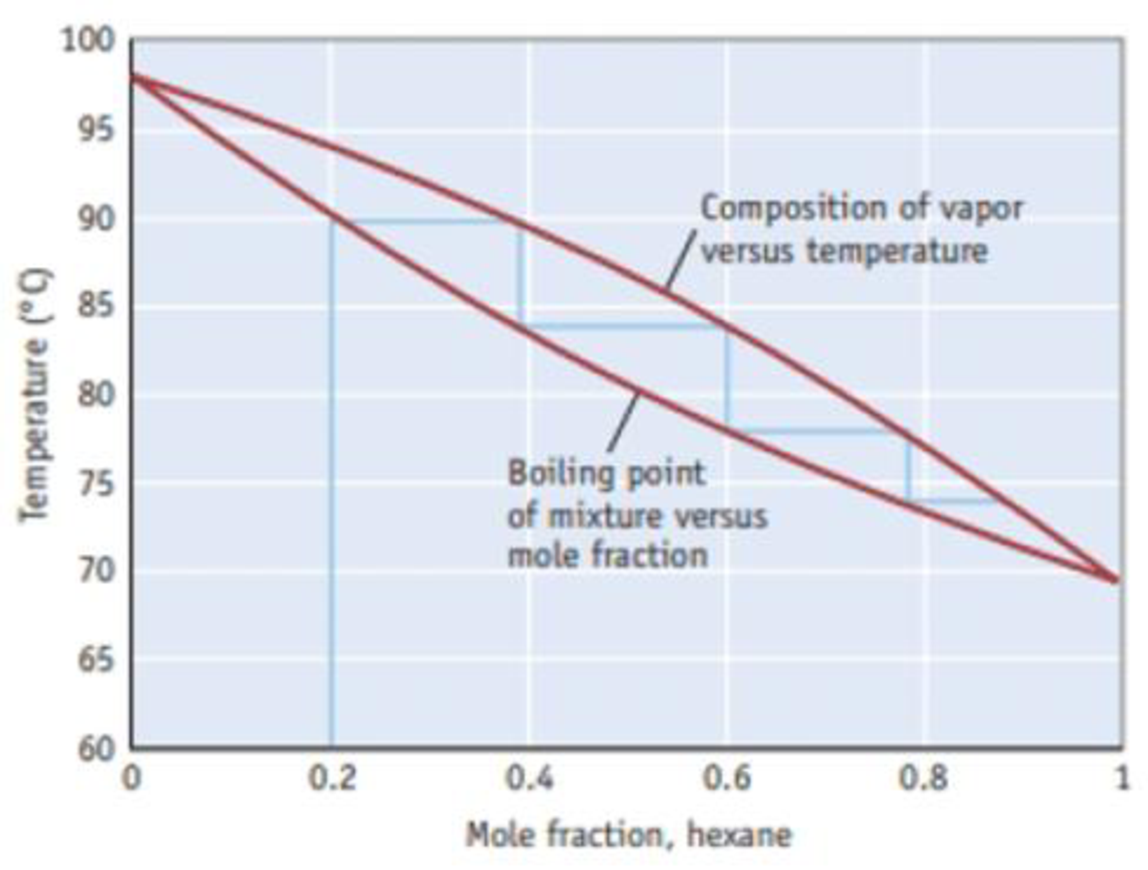Chapter 13.5, Problem 1.2ACP

Chapter
Section
Textbook Problem

How many theoretical plates are required to produce a solution with a mole fraction of hexane greater than 0.90?Figure B A temperature-composition diagram for hexane (C6H14)-heptane (C7H16) mixtures.

Interpretation Introduction

Interpretation: By using the given temperature-composition diagram it should be determined that how many theoretical plates are required to produce a solution with a mole fraction of hexane greater than 0.90.

Concept introduction:

• Mole fraction is one of the concentrations expressing term which is equal to the number of moles of a component divided by the total number of a solution. The mole fraction of all components of a solution, when added together will equal to 1

The mole fraction of component a can be determined by using the equation,

Molefractionofa(Xa)=MoleofaTotalmoles

• Fractional distillation it is a separation process in which the volatile components of a mixture are split from one another by heating the mixture in a column and collecting and condensing the vapors drawn from different levels of the column.
• The efficiency of fractional distillation column is expressed in terms of theoretical plate. Each evaporation-condensation cycles requires one theoretical plate. The greater the number of theoretical plates the better the separation for most mixtures.
Explanation

Temperature – composition diagram for hexane (C6H14) – heptane (C7H16) mixtures.

Here the lower curved line indicates the boiling point of the mixture as a function of mole fraction of the more volatile component, hexane. The upper curve indicates the mole fraction of hexane in the vapor phase above the boiling solution. The blue line represents the change in composition of the mixture in the course of the fractional distillation of a mixture with an initial hexane mole fraction of 0

Still sussing out bartleby?

Check out a sample textbook solution.

See a sample solution

The Solution to Your Study Problems

Bartleby provides explanations to thousands of textbook problems written by our experts, many with advanced degrees!

Get Started

Chylomicrons, a class of lipoprotein, are produced in the live. T F

Nutrition: Concepts and Controversies - Standalone book (MindTap Course List)

What is the structure and function of the extracellular matrix?

Biology: The Dynamic Science (MindTap Course List)

When alcohol is rubbed on your body, it lowers skin temperature. Explain this effect.

Physics for Scientists and Engineers, Technology Update (No access codes included)